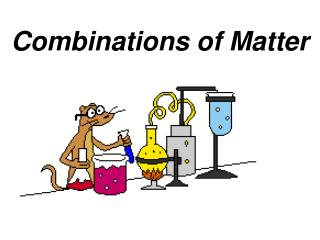DownloadDownload PresentationCombinations of Matter

Combinations of Matter

Télécharger la présentationCombinations of Matter

- - - - - - - - - - - - - - - - - - - - - - - - - - - E N D - - - - - - - - - - - - - - - - - - - - - - - - - - -
Presentation Transcript

1. Combinations of Matter

2. SIGNS OF CHEMICAL RXNS Color change - new substances might reflect different colors of light. Heat change - the container may get hotter or colder. Gas produced - bubbles or smoke may be observed. Precipitate formed - an insoluble solid might form in a liquid.

3. CONDITION FOR A CHEMICAL RXN: • reactants have ability to form chemical bonds. • Bonds are formed by valence electrons of reactant atoms.

4. “If valence electrons of substances brought together do not match to complete the Octet Rule, there will be no chemical reaction”.

5. Parts of a rxn:

6. Reactants  Products

7. Chemical Equations

8. 1 WORD EQUATION Copper oxide (ore) plus hydrogen gas produce copper metal plus water

9. 2 CHEMICAL EQUATION CuO + H2 Cu + H2O

10. Coefficient Subscript Element 5H2

11. Reactants Products CuO + H2--> Cu + H2O BALANCED EQUATION 1 copper 1oxygen  2 hydrogen 1 copper 2 hydrogen 1 oxygen

12. Reactants Products 2 H2 + O2--> BALANCED EQUATION

13. Reactants Products __H2O __H2 +__ O2--> BALANCED EQUATION

14. Reactants Products 2 H2 + O2--> 2 H2O BALANCED EQUATION 4 hydrogen2 oxygen  4 hydrogen 2 oxygen

15. Chemical Equation:a way to represent chemical reactions on paper. 2 H2 + O2--> 2 H2O

16. Law of conservation of mass: “During a chemical rxn, atoms are neither created nor destroyed. The number of atoms remains constant throughout the reaction. Since the number of atoms doesn't change, the mass must remain constant as well”.

17. Equations must be balanced because:Atoms can be neither created nor destroyed in an ordinary chemical reaction, so there must be the same number of atoms on both sides of the equation.

18. Balance equations by changing coefficientsnever by changing formula subscripts

19. Interactive Tutorials on Balancing Equations GenChem - Balance Equation Click on this site: http://www.wfu.edu/~ylwong/balanceeq/balanceq.html

20. FLASHBULBS

21. When a flashbulb is set off, Magnesium combines with oxygen to form Magnesium oxide.

22. Mg + O2

23. Chemical reaction types: synthesis, decomposition, single replacement, double replacement, andcombustion.

24. A + B C C A + B A + BX AX + B AX + BYAY + BX

25. Example: element + element   compound A + B C

26. 2Na + Cl22NaCl 4Fe + 3O22Fe2O3

27. REACTION OF COPPER POWDER • WORD EQUATION • CHEMICAL EQUATION • BALANCED CHEMICAL • EQUATION

28. REACTION OF COPPER POWDER 2Cu + O2 (2CuO) Copper Oxide (2CuO) Pesticide Fungicide

29. compound   element + element CA + B 2H2O   2H2 + O2 H2CO3H2O + CO2

30. Single replacement: a single element replaces an element in a compound. element + compound   compound + element Zn + 2HCl   ZnCl2 + H2 2Na + 2H2O   2NaOH + H2

31. Results: Complete the table below.

32. Double replacement:two different atoms in two different compounds exchange places. compound + compound   compound + compound MgCO3 + 2HCl   MgCl2 + H2CO3 2KCl + Pb(NO3)2  2KNO3 + PbCl2

33. Combustion When oxygencombines with another compound to form water and carbon dioxide. Reaction is exothermic (produce heat). e.g. burning of napthalene C10H8 + 12 O2 10 CO2 + 4H2O

34. SUMMARY: TYPES OF REACTIONS 2Na + Cl22NaCl MgCO3 + 2HCl    MgCl2 + H2CO3 H2CO3H2O + CO2 Zn + 2HCl    ZnCl2 + H2

35. A + B C C A + B A + BX AX + B AX + BYAY + BX